Sort

By default Sort arranges items in cannonical order rather than numerical
order. This is reasonable because some or all of the elements may not have a
numeric value. If all elements of a list have the heads Integer, Rational, or
Real the cannanocal ordering is a numerical ordering. In the next cell some
elements of the list don't have the head Integer, Rational or Real and the
elements aren't sorted numerically even though they could be sorted
numerically.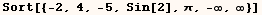You can provide Sort a second argument which specifies how elements should be
compared when they are sorted. In the next cell Less is used as an ordering
function and the list is sorted numerically. Of course you would get the list
in opposite order if the ordering function Greater was used. However, Allan
Hayes has pointed out in the MathGroup that Sort is much slower when an
ordering function is specified.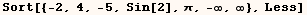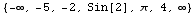The ordering function (#1<#2&) has the same effect as the ordering  function Less, but is more cryptic. In this case there isn't a good reason to  use the more cryptic ordering function, but in more complicated cases the  cryptic notation is harder to avoid. If you aren't familiar with the #&  notation see the section on Function.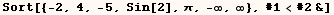Suppose you have the matrix created by the next cell and you want to sort the
rows according to the value in a certain row of the matrix. The next cell
makes such a matrix we can use as an example.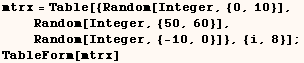6 52 -9 6 53 -2 9 57 -3 4 50 -7 8 53 -7 3 56 -7 7 55 -8 10 56 -5

In the next cell the matrix is sorted according to the value in the first
column.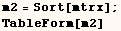3 56 -7 4 50 -7 6 52 -9 6 53 -2 7 55 -8 8 53 -7 9 57 -3 10 56 -5

If we want to sort the matrix according to the value in the second column we
can use the ordering function  (#1[]<#2[]&) as in the next cell.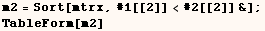4 50 -7 6 52 -9 8 53 -7 6 53 -2 7 55 -8 10 56 -5 3 56 -7 9 57 -3

The code in the next cell effectively sorts the matrix on the second  column. In this case each row is rotated before using Sort. Then Sort can be  used without an ordering function. After the matrix is sorted the columns are  put back in the original order. Sort evaluates much faster this time because an ordering function wasn't specified. When given a  matrix with thousands of rows this method is about 18 times faster even after  accounting for the time to rotate each column before and after sorting.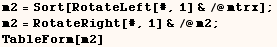4 50 -7 6 52 -9 8 53 -7 6 53 -2 7 55 -8 3 56 -7 10 56 -5 9 57 -3

Sort can work with any expression. In the next cell Sort takes an expression